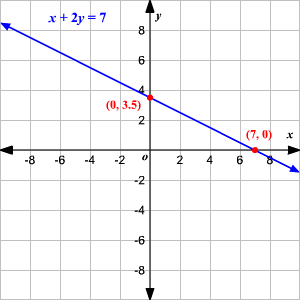Question

# Describe Graphing Linear Equations

Open in App
Solution

## The graph of a linear equation in two variables is a line (that's why they call it linear ).If you know an equation is linear, you can graph it by finding any two solutions$\left({x}_{1},{y}_{1}\right)$ and $\left({x}_{2},{y}_{2}\right)$ plotting these two points, and drawing the line connecting them.For example, equation $x+2y=7$ find two solutions, corresponding to the $x$ -intercepts and $y$ -intercepts of the graph, by setting first $x=0$ and then $y=0$ When $x=0$ we get:$\begin{array}{l}0+2y=7\\ y=3.5\end{array}$When $y=0$ we get:$\begin{array}{l}x+2\left(0\right)=7\\ x=7\end{array}$So the two points are $\left(0,3.5\right)$ and $\left(7,0\right)$ Plot these two points and draw the line connecting them.Suggest Corrections1Similar questionsRelated VideosMATHEMATICS
Watch in AppExplore more